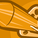# New to Qlik Sense

If you’re new to Qlik Sense, start with this Discussion Board and get up-to-speed quickly.

Announcements
Qlik Insider: SaaS capabilities for Data Integration and Analytics, May 25th! Register Now
cancel
Showing results for
Did you mean:Partner

## top 5 bottom 5 syntax in expression

I've created 2 buttons which is Top 5 and Bottom 5 (using a variable). I have my expression created and it works for the most part. The problem is that it shows me 4 bars for top 5 and 3 bars for bottom 5. I was expecting it to give me 5 bars, hence the <=5 placed in the expression. Any ideas?

If(vTopBot=1,

Sum({< merchandise ={"=Rank(Sum( COST))<=5 "}>} COST ),
Sum({< merchandise ={"=Rank(-Sum( COST))<=5"}>} COST )

)

1 Solution

Accepted SolutionsSpecialist III

if(aggr(rank(sum(COST)),merchandise )<=5,merchandise )

this will give top5 merchandise

Channa
4 RepliesSpecialist III

if(aggr(rank(sum(COST)),merchandise )<=5,merchandise )

this will give top5 merchandise

ChannaPartner
Author

Thank you. If I wanted to put both top and bot in the same formula would I do something like -

if(aggr(rank(sum(COST)),merchandise )<=5,merchandise ), aggr(rank(-sum(COST)),merchandise )<=5,merchandiseSpecialist III

if(aggr(rank(sum(COST)),merchandise )<=5 or  aggr(rank(-sum(COST)),merchandise )<=5,merchandise )

try this

ChannaSpecialist III

=aggr(if(rank(sum(COST))<=5 or rank(-sum(COST))<=5,merchandise ),merchandise )

it should work

i am using this bro..

below one works for me

=aggr(if(rank(sum(CUSTOMS_VALUE_QAR))<=5 or rank(-sum(CUSTOMS_VALUE_QAR))<=5,COUNTRY_NAME_EN),COUNTRY_NAME_EN)

Channa